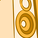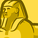# New to Qlik Sense

If you’re new to Qlik Sense, start with this Discussion Board and get up-to-speed quickly.

Announcements
cancel
Showing results for
Did you mean:Contributor III

## Filter selection issue with Multiple if statement

I have written multiple if statements in two ways to create a master measure.

way 1:

pick(match(Account,'MTDAvgAUM','MTDEndAUM','YTDAvgAUM','YTDEndAUM'),Sum({<Account={'MTDAvgAUM'}, [Period Date]={"\$(=Max([Period Date]))"}, Period=, [Fiscal Month]=, [Fiscal Quarter]=, [Fiscal Year]=>} [Amount])
,Sum({<Account={'MTDEndAUM'}, [Period Date]={"\$(=Max([Period Date]))"}, Period=, [Fiscal Month]=, [Fiscal Quarter]=, [Fiscal Year]=>} [Amount])
,Sum({<Account={'YTDAvgAUM'}, [Period Date]={"\$(=Max([Period Date]))"}, Period=, [Fiscal Month]=, [Fiscal Quarter]=, [Fiscal Year]=>} [Amount])
,Sum({<Account={'YTDEndAUM'}, [Period Date]={"\$(=Max([Period Date]))"}, Period=, [Fiscal Month]=, [Fiscal Quarter]=, [Fiscal Year]=>} [Amount]))

way 2:

Num(
If(Account='MTDAvgAUM', Sum({<Account={'MTDAvgAUM'}, [Period Date]={"\$(=Max([Period Date]))"}, Period=, [Fiscal Month]=, [Fiscal Quarter]=, [Fiscal Year]=>} [Amount])
,If(Account='MTDEndAUM', Sum({<Account={'MTDEndAUM'}, [Period Date]={"\$(=Max([Period Date]))"}, Period=, [Fiscal Month]=, [Fiscal Quarter]=, [Fiscal Year]=>} [Amount])
,If(Account='YTDAvgAUM', Sum({<Account={'YTDAvgAUM'}, [Period Date]={"\$(=Max([Period Date]))"}, Period=, [Fiscal Month]=, [Fiscal Quarter]=, [Fiscal Year]=>} [Amount])
,If(Account='YTDEndAUM', Sum({<Account={'YTDEndAUM'}, [Period Date]={"\$(=Max([Period Date]))"}, Period=, [Fiscal Month]=, [Fiscal Quarter]=, [Fiscal Year]=>} [Amount]),
sum(Amount))))),'#,##0')

But problem is when i am selecting account =MTDAvgAUM in filter , for rest of the if clause it giving 0 which i dont want to show. but i dont want to uncheck include zero value option also.

I want to show only result for selected account.

Thanks

Labels (3)

• ### set analsyis

1 Solution

Accepted SolutionsPartner

Since you have same set analysis for the 4 accounts and the only difference is the account itself (which the chart will handle it automatically if the dimension that you are using in the chart is "Account") then I think the below should word

Num(If(MAtch(Account,'MTDAvgAUM','MTDEndAUM','YTDAvgAUM','YTDEndAUM'),Sum({<[Period Date]={"\$(=Max([Period Date]))"}, Period=, [Fiscal Month]=, [Fiscal Quarter]=, [Fiscal Year]=>} [Amount]),sum(Amount)),'#,##0')

Yousef Amarneh
6 RepliesPartner

can you share a screenshot from the chart/table

Yousef AmarnehContributor III
AuthorPartner

Since you have same set analysis for the 4 accounts and the only difference is the account itself (which the chart will handle it automatically if the dimension that you are using in the chart is "Account") then I think the below should word

Num(If(MAtch(Account,'MTDAvgAUM','MTDEndAUM','YTDAvgAUM','YTDEndAUM'),Sum({<[Period Date]={"\$(=Max([Period Date]))"}, Period=, [Fiscal Month]=, [Fiscal Quarter]=, [Fiscal Year]=>} [Amount]),sum(Amount)),'#,##0')

Yousef AmarnehContributor III
Author

thanks a lot for help. It worked.Contributor III

Hi Yousef,

I have similar issues. I am using the below expression in a line chart. I get the trend line if I select only one channel, however if i select multiple channels, the chart is simply blank. pl help me solve this.

if(Channel='AMS',Sum({\$<Variables={"ams_direct_cost"}>}Values),
if(Channel='GCM',Sum({\$<Variables={"gcm_direct_cost"}>}Values),
if(Channel='FB',Sum({\$<Variables={"fb_direct_cost"}>}Values),
if(Channel='ISG',Sum({\$<Variables={"isg_direct_cost"}>}Values)))))

I also tried pick match combo which also didn't yield any result. Can u pl help me fix this.

Pick(match('AMS','GCM','FB','ISG'),
Sum({\$<Variables={"ams_direct_Impressions"}>}Values),
Sum({\$<Variables={"gcm_direct_Impressions"}>}Values),
Sum({\$<Variables={"fb_direct_Impressions"}>}Values),
Sum({\$<Variables={"isg_direct_Impressions"}>}Values))Specialist

You can also try this solution

Sum({<Account=p(Account), [Period Date]={"\$(=Max([Period Date]))"}, Period=, [Fiscal Month]=, [Fiscal Quarter]=, [Fiscal Year]=>} [Amount])